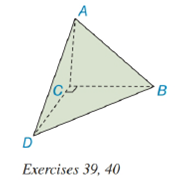Chapter 9.2, Problem 39EElementary Geometry For College St...

7th Edition
Alexander + 2 others
ISBN: 9781337614085

Solutions

Chapter
SectionElementary Geometry For College St...

7th Edition
Alexander + 2 others
ISBN: 9781337614085
Textbook Problem

Exercise 39 and 40 are based upon the “uniqueness of volume.”A tetrahedron (not rectangular) has vertices at A , B , C , and D . The length of the altitude from A to the base ( △ B C D ) measures 6 i n . It is given that m ∠ B C D = 90 ° , B C = 4 i n . , and C D = 8 i n . a) Find the volume of the pyramid.b) Find the length of the altitude from vertex D to the base ( △ A B C ); note that A A B C = 12 i n 2 .To determine

(a)

To find:

The volume of the pyramid.

Explanation

Given:

A tetrahedron (not rectangular) has vertices at A, B, C, and D. The length of the altitude from A to the base (BCD) measures 6in. It is given that mBCD=90°, BC=4in., and CD=8in. as below,

Properties Used:

The volume V of a pyramid having a base area B and an altitude of length h is given by V=13Bh.

Area of a right-angled triangle is 12×base×height.

Approach Used:

i) Calculate the area of the base triangle.

ii) Substitute the values of area B and the height h in equation V=13Bh to find the value of volume V.

Calculation:

From the given figure

the altitude is h=6in. Considering the base triangle BCD, having mBCD=90°, BC=4in

To determine

(b)

To find:

The length of the altitude from vertex D to the base (ABC).

Still sussing out bartleby?

Check out a sample textbook solution.

See a sample solution

The Solution to Your Study Problems

Bartleby provides explanations to thousands of textbook problems written by our experts, many with advanced degrees!

Get Started

Find more solutions based on key concepts